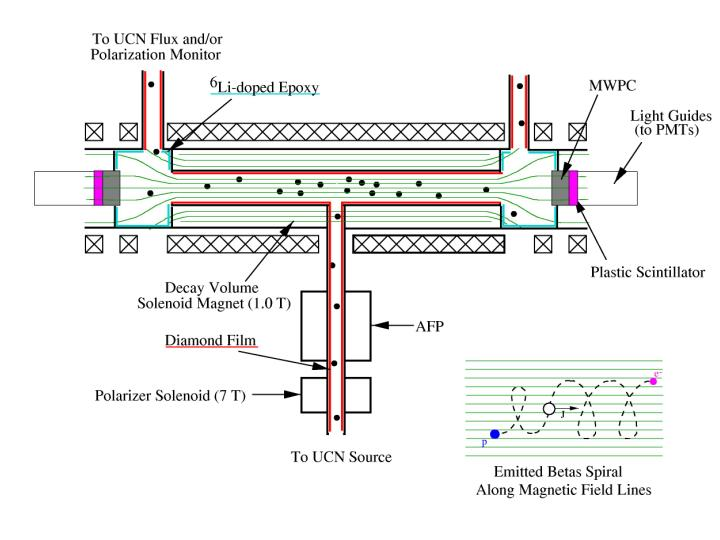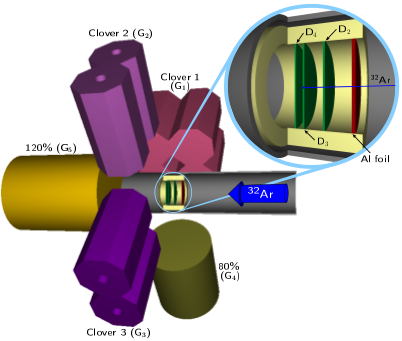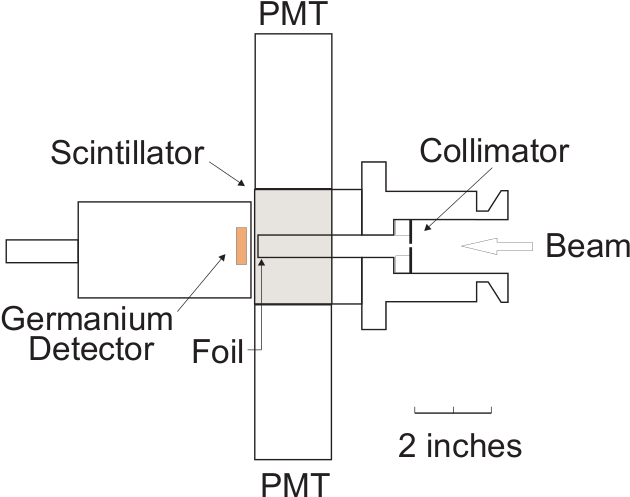## Beta-neutrino angular correlation in 6HePeople:

Peter Mueller, Zheng-Tian Lu, William Williams
Argonne National Lab
Brent Delbridge, Alejandro Garcia, Greg Harper, Andreas Knecht, Hamish Robertson, Erik Swanson, Satoshi Utsuno, Doug Will, Chris Wrede, David Zumwalt, Ran Hong
University of Washington

Short description:

Studies of nuclear beta decay have a long standing history in testing the Standard Model of particle physics. With the dominant (V-A) structure of the weak interaction determined, measurements of the angular correlation between the electron and neutrino momenta in nuclear beta decay can be used to search for scalar and tensor contributions to the weak interaction. The current best measurement on the electron-neutrino angular correlation coefficient in the decay of 6He dates back to 1963 by measuring the energy spectrum of the recoiling 6Li nucleus and amounts to -0.3343 +/- 0.0030. Its compatibility with the Standard Model expectation allows to constrain tensor contributions to (|CT|2+|C'T|2)/(|CA|2+|C'A|2) < 0.4%.
We intend to improve on this measurement by confining 6He atoms in a magneto-optical trap and detecting the recoiling nucleus and emitted electron in coincidence. The foreseen sensitivity in the measurement of the angular correlation coefficient will be approximately 0.1%.
The experiment takes place here at CENPA where we use our tandem accelerator to produce 6He via the 7Li(d,3He)6He reaction. The magneto-optical trap has been developed by the group at Argonne National Lab and has already successfully been used to trap 6He and 8He to measure their charge radii.

## UCNA: Beta asymmetry in neutron decayPeople:

UCNA collaboration
LANL, NCSU, Caltech, UW, VirginiaTech,
A. Saunders and A. Young (spokepersons)

Recent Publications:

First Measurement of the Neutron β Asymmetry with Ultracold Neutrons
R. W. Pattie, Jr. et al. (UCNA Collaboration), Phys. Rev. Lett. 102, 012301 (2009)

Determination of the Axial-Vector Weak Coupling Constant with Ultracold Neutrons
J. Liu et al. (UCNA Collaboration), Phys. Rev. Lett. 105, 181803 (2010)

Short description:

The UCNA collaboration constructed an apparatus to measure the beta asymmetry parameter A in neutron decay. The experiment is located at the Los Alamos Neutron Science Center (LANSCE) providing the ultracold neutrons used in this apparatus.
Polarized ultracold neutrons are filled into a storage volume within a strong magnetic field. Two electron detectors at both ends of the chamber are used to directly measure the electron decay asymmetry with respect to the magnetic field direction.
The goal of the experiment is to extract the ratio of axial to vector coupling constants gA/gV from the measured decay asymmetry with a precision of about 0.2%. With the neutron lifetime as an additional input, it is then possible to calculate Vud, the up-down element of the weak quark mixing matrix. All these values are basic elements of the Standard Model. In addition, comparing these results to measurements of nuclear beta decay serves as a stringent test of our current understanding.

## Scalar interactions: 32ArPeople:

Varied list: lots of experiments

Short Description:

In 1999, we measured the beta-neutrino correlation in the decay of 32Ar at CERN-ISOLDE to search for scalar currents that would indicate physics beyond the standard model. The experimental value for the correlation coefficient was consistent with the standard-model prediction and was used set a limit on scalar-current contributions to nuclear beta decay that is still the second-most precise limit of its kind. Since then, we have pursued a variety of related experiments. For example, we have re-measured the superallowed T= 2 beta decay of 32Ar at the NSCL at Michigan State University in order to determine the ft value of this decay and check the isospin symmetry breaking corrections used to calculate the CKM matrix element Vud from T =1 beta decays. In addition, we have measured the mass of 31S with the Canadian Penning Trap at Argonne National Laboratory and the mass of 32Cl with the Q3D Magnetic Spectrograph at the Maier Leibnitz Laboratory in Garching, Germany. These mass measurements affect the results of both 32Ar beta-decay experiments (because they affect the QEC value for the decay) and contribute to the most precise test of the isobaric multiplet mass equation in nuclei. Currently, we are investigating techniques that might lead to 32Ar beta-decay measurements with improved precision.

## Double beta decay matrix elements: 100Tc and 116CdPeople:

A. Garcia (CENPA/UW), S. Sjue (now at TRIUMF), E. Swanson (CENPA/UW), C. Wrede (CENPA/UW), D. Melconian (now at Texas A&M University, College Station, TX 77843, USA), A. Algora (Instituto de Fisica Corpuscular, University of Valencia, Valencia, Spain), I. Ahmad (Argonne) + IGISOL group at Jyvaskyla (~10+ people)

Short description:

Both 100Mo and 116Cd are zero-neutrino double-beta-decay candidates with Jpi =0+. Because the ground states of the respective intermediate nuclei 100Tc and 116In have Jpi = 1+, the contributions of the virtual transitions through the ground states are relevant to the corresponding two-neutrino double-beta decays. In fact, they nearly reproduce the measured two-neutrino double-beta decay halflives. Consequently, one can use the beta-decay matrix elements for the intermediate nuclei along with the corresponding two-neutrino double-beta-decay matrix elements to provide a relatively stringent test of nuclear models that are used to calculate zero-neutrino double beta decay matrix elements. The electron-capture (EC) decay of 100Tc can also be used to determine the efficiency of a potential neutrino detector. However, the experimental determinations of the EC decay branches of interest are challenging because they are only small fractions of the main decays and co-produced radioactivities contribute significant background counts in the regions of interest. We have developed a detection system at CENPA for measuring EC decays in Jyvaskyla, Finland, where we have taken advantage of the high-purity beams produced at the IGISOL radioactive ion beam facility in combination with the JYFLTRAP Penning trap to achieve significant improvements in the determinations of the EC branches of 100Tc and 116In.

## emiT: search for Time-Reversal Symmetry Breaking in neutron beta decayNews (February 2011): after much work on analysis emiT is close to publishing new results.

Publications

New limit on the D coefficient in polarized neutron decay,

L.J. Lising et al., Phys. Rev. C 62, 055501 (2000).

emiT: An apparatus to test time reversal invariance in polarized neutron decay
H.P. Mumm et al., Rev. Sci. Instruments 75, 5343 (2004).